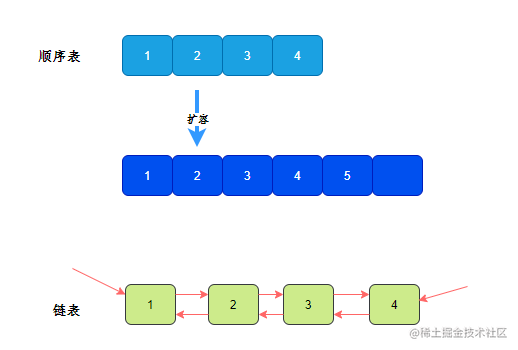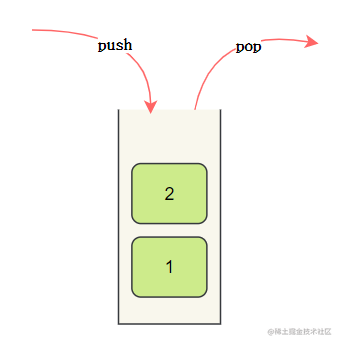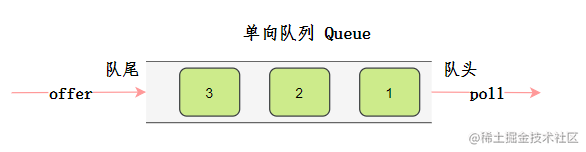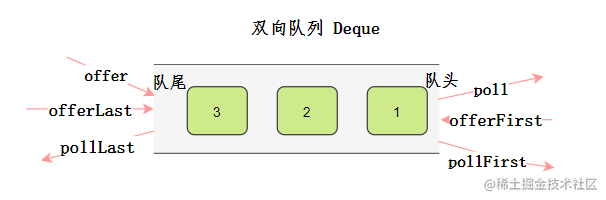# 集合

String字符串charAt toCharArray split substring indexOf lastIndexOf replace length
Stackpush pop peek isEmpty size
Queue队列offer poll peek isEmpty size
Deque双向队列offerFirst offerLast pollFirst pollLast peekFirst peekLast isEmpty size
PriorityQueue优先队列offer poll peek isEmpty size
Setadd remove contains isEmpty size first(TreeSet) last(TreeSet)
Mapput get getOrDefault containsKey containsValue keySet values isEmpty size

## 数组

### Arrays

Arrays是比较常用的数组工具类，可以完成排序、拷贝等功能。

• 从小到大排序：Arrays.sort(int[] arr)` `Arrays.sort(int[] arr, int fromIndex, int toIndex)
``````Arrays.sort(int[] arr, int fromIndex, int toIndex, 比较器);   //一定是需要泛型

Arrays.sort(arr, (o1, o2) -> o2 - o1);   //数组全部 从大到小排序 跟Collections.sort()一样

Arrays.sort(arr, 0, 3, (o1, o2) -> o2 - o1);   //从大到小排序，只排序[0, 3)

• 拷贝：Array.copyOf
``````int[] a = new int;
int[] newA = Array.copyOf(a, 5);

## 列表### 基本方法

• 构造
``````List<Integer> array = new ArrayList<>();    // 顺序表
// Set<Integer> a = new HashSet....
List<Integer> b = new ArrayList<>(a);     //接受一个集合容器

• get
``````get(int index)    // 返回元素位置在index的元素e --- array O(1), list O(n)

• #### size

``````size()    // 返回数组/链表长度 --- O(1)

``````add(E e)    // 在尾部添加一个元素e --- O(1)
add(int index, E e)    // 在index位置插一个元素e --- O(n)

• remove
``````.remove(int index)    // 删除位于index的元素，并返回删除元素e --- 删除最后元素为O(1)， 其余为O(n)
//删除最后元素 list.remove(list.size() - 1);

• subList
``````.subList(int from, int to)    // 相当于返回原数组的一个片段,但不要对其进行改动，改动会影响原数组 --- O(1)
// List<Integer> list, 对原来的list和返回的list做的“非结构性修改”(non-structural changes)，
//都会影响到彼此对方. 如果你在调用了sublist返回了子list之后，如果修改了原list的大小，那么之前产生的子list将会失效，变得不可使用

### 集合工具

Collections是集合工具类，提供了一些操作集合的方法。

• 排序
``````Collections.sort(list); 从小到大排序
Collections.sort(list, (o1, o2) -> o2 - o1); 从大到小排序， 第二个参数为一个比较器

get(int index)O(1)O(n)，平均 n / 4步
add(int index, E element)O(n) ,平均n / 2步O(n)，平均 n / 4步
remove(int index)O(n) 平均n /2步O(n)，平均 n / 4步

## 栈

Java中定义了`Stack`接口，实现类是`Vector``Vector`是一个祖传集合类，不推荐使用。

`Deque`接口实现了完善堆栈操作集合，它有一个我们熟悉的实现类`LinkedList`• 构造
``````Deque<Integer> stack=new LinkedList<>();

• push
``````push(E e);    // 入栈元素e， 返回值为元素e --- O(1)

• pop
``````.pop();    // 出栈一个元素，返回出栈元素e --- O(1)

• peek
``````peek();    // 查看栈顶元素， 返回值为栈顶元素e --- O(1)

• isEmpty
``````isEmpty()    // 若栈空返回true， 否则返回false --- O(1)

• size
``````size()    // 返回栈中元素个数 --- O(1)

## 队列• 构造
``````Queue<Integer> q = new LinkedList<>();

• offer
``````offer(E e);    // 队尾加入元素e。 若成功入队返回值true，否则返回false --- O(1)

• poll
``````poll();    // 出队头，返回出队元素e --- O(1)

• peek
``````peek();    // 查看队头元素， 返回值队首元素e --- O(1)

• isEmpty
``````isEmpty()    // 若队空返回true， 否则返回false --- O(1)

• size
``````size()    // 返回队中元素个数 --- O(1)

## 双向队列

`Queue`有一个子接口`Dueue`，即双向队列，和单向队列不同，它的出队入队可以从两个方向。• 构造
``````Dueue<Integer> q = new LinkedList<>();

• offFirst
``````offFirst(Object e)   // 将指定元素添加到双端队列的头部 --- O(1)

• offLast
``````offLast(Object e)   //将指定元素添加到双端队列的尾部 --- O(1)

• pollFirst
``````pollFirst()   //获取并删除双端队列的第一个元素 --- O(1)

• pollLast
``````pollLast()   //获取并删除双端队列的最后一个元素 --- O(1)

• peekFirst
``````peekFirst()   //获取但不删除双端队列的第一个元素 --- O(1)

• peekLast
``````peekLast()   //获取但不删除双端队列的最后一个元素 --- O(1)

## 优先队列

• 构造
• 小根堆
``````Queue<Integer> minH = new PriorityQueue<>();    // 小根堆，默认大小为11 相当于  new PriorityQueue<>(11)
Queue<Integer> minH = new PriorityQueue<>(100);  // 定义一个默认容量有100的小根堆。在当中增加元素会扩容，只是开始指定大小。不是size，是capacity

• 大根堆
``````Queue<Integer> maxH = new PriorityQueue<>((i1, i2) -> i2 - i1);    // 大根堆，默认大小为11 相当于  new PriorityQueue<>(11, (i1, i2) -> i2 - i1)
Queue<Integer> maxH = new PriorityQueue<>(100, (i1, i2) -> i2 - i1);    // 定义一个默认容量有100的大根堆。在当中增加元素会扩容，只是开始指定大小

• offer
``````offer(E e);    // 在堆中加入元素e，并调整堆。若成功入堆返回值true，否则返回false --- O(logN)

• poll
``````poll();    // 弹出堆顶元素，并重新调整堆，返回出队元素e --- O(logN)

• peek
``````peek();    // 查看堆顶元素， 返回值堆顶元素e --- O(1)

## 散列表

• 构造
``````Map<Characters, Integer> map = new HashMap<>();

• put
``````put(K key, V value);    // 在Map中加入键值对<key, value>。返回value值。如果Map中有key，则replace旧的value --- O(1)

• get
``````get(K key);    // 返回Map中key对应的value。若Map中没有该key，则返回null --- O(1)

• getOrDefault
``````getOrDefault(K key, V defaultValue);    // 返回Map中key对应的value。若Map中没有该key，则返回defaultValue --- O(1)

// For example:
// Map<Character, Integer> map = new HashMap<>();
// if (...)    // 如果发现k，则k在Map中的值加1。没一开始没有k，则从0开始加1。（相当于给了key在Map中的一个初试值）
map.put('k', map.getOrDefault('k', 0) + 1);

• containsKey
``````containsKey(Key key);    // 在Map中若存在key，则返回true，否则返回false --- O(1)

get(x) == null // 可以代替改用法

• keySet
``````keySet();    // 返回一个Set,这个Set中包含Map中所有的Key --- O(1)

// For example:
// We want to get all keys in Map
// Map<Character, Integer> map = new HashMap<>();
for (Character key : map.keySet()) {
// Operate with each key
}

• values
``````values();    // 返回一个Collection<v>,里面全是对应的每一个value --- O(1)

// For example:
// We want to get all values in Map
// Map<Character, Integer> map = new HashMap<>();
for (Integer value : map.values()) {
// Operate with each values
}

• isEmpty
``````isEmpty()    // 若Map为空返回true， 否则返回false --- O(1)

• size
``````.size()    // 返回Map中中键值对<K, V>的个数 --- O(1)

## Set

Set是一种没有重复元素的集合，常用的实现是`HashSet`

• 构造
``````Set<Integer> set = new HashSet<>();
List<Integer> list = new ArrayList<>....;
Set<Integer> set = new HashSet<>(list);

``````.add(E e);    // 在集合中添加元素E e， 若成功添加则返回true，若集合中有元素e则返回false --- O(1)

• remove
``````remove(E e);    // 在集合中删除元素e，若删除成功返回true；若集合中没有元素e，返回false --- O(1)

• contains
``````contains(E e);    // 若存在元素e，则返回true，否则返回false --- O(1)

• isEmpty
``````isEmpty()    // 若集合为空返回true， 否则返回false --- O(1)

• size
``````size()    // 返回集合中中元素个数 --- O(1)

• first
``````first()    // 返回集合里的最小值（若给了比较器从大到小则是返回最大值）

• last
``````last()    // 返回集合里的最大值（若给了比较器从大到小则是返回最小值）

## 字符串

### String• 初始化

``````String s = ``"abc"``;

``````// s = "abc"``String s2 = ``new` `String(s);

``````// s = "abc";
// char[] c = s.toCharArray();
String s3 = new String(c);

// 可以偏移
// public String(char value[], int offset, int count)
String s4 = new String(c, 1, 2);    // [offset, offset + count) [)

// 把char[] 变成字符串
char[] ch = {'a', 'b', 'c'};
String.valueOf(ch);

• charAt
``````charAt(int index);    // 返回index位置的char --- O(1)

• length
``````length();    // 返回字符串长度 --- O(1)

• substring
``````substring(int beginIndex, int endIndex);    // 返回字符片段[beginIndex, endIndex) --- O(n)

substring(int beginIndex);    // 返回字符片段[beginIndex, end_of_String) 就是从beginIndex开始后面的 ---- O(n)

• indexOf
``````indexOf(String str)    // 返回str第一个出现的位置(int)，没找到则返回-1。 --- O(m * n) m为原串长度， n为str长度
// (假如要找一个字符char c，str可以表示成String.valueOf(c),然后作为参数传进去.

s.indexOf(String str, int fromIndex);    // 同上，但从fromIndex开始找 --- O(m * n)

• lastIndexOf
``````lastIndexOf(String str);    // 返回str最后出现的位置(int)，没找到则返回-1。 --- O(m * n) m为原串长度， n为str长度
// (假如要找一个字符char c，str可以表示成String.valueOf(c),然后作为参数传进去.

lastIndexOf(String str, int fromIndex);    // 同上，
//但从fromIndex开始从后往前找 [0 <- fromIndex] --- O(m * n)

• replace
``````replace(char oldChar, char newChar);    // 返回一个新字符串String，其oldChar全部变成newChar --- O(n)

• toCharArray
``````toCharArray();   // 返回char[] 数组。 把String编程字符数组 --- O(n)

• trim
``````trim();    // 返回去除前后空格的新字符串 --- O(n)

• split
``````split(String regex);    // 返回 String[]，以regex(正则表达式)分隔好的字符换数组。 ---- O(n)

// For example
// 从非"/"算起 若"/a/c" -> 会变成"" "a" "c"
String[] date = str.split("/");     // date:1995 date:12 date:18 --- O(n)

• toLowerCase, toUpperCase
``````s = s.toLowerCase();    // 返回一个新的字符串全部转成小写 --- O(n)
s = s.toUpperCase();    // 返回一个新的字符串全部转成大写 --- O(n)

### StringBuilder

• 构造
``````StringBuilder sb = new StringBuilder();

• charAt
``````charAt(int index);    // 返回index位置的char --- O(1)

• length
``````length();    // 返回缓冲字符串长度 --- O(1)

• apped
``````append(String str)   // 拼接字符串 --- O(n)

• toString
``````toString();    // 返回一个与构建起或缓冲器内容相同的字符串 --- O(n)

# 数学

## 最大最小值

``````fmax = Float.MAX_VALUE;

fmin = Float.MIN_VALUE;

dmax = Double.MAX_VALUE;

dmin = Double.MIN_VALUE;

bmax = Byte.MAX_VALUE;

bmin = Byte.MIN_VALUE;

cmax = Character.MAX_VALUE;

cmin = Character.MIN_VALUE;

shmax = Short.MAX_VALUE;

shmin = Short.MIN_VALUE;

imax = Integer.MAX_VALUE;

imin = Integer.MIN_VALUE;

lmax = Long.MAX_VALUE;

lmin = Long.MIN_VALUE;

## Math

• max
``````Math.max(long a, long b);    //返回两个参数中较大的值

• sqrt
``````Math.sqrt(double a);    //求参数的算术平方根

• abs
``````Math.abs(double a);  //返回一个类型和参数类型一致的绝对值

• pow
``````Math.pow(double a, double b);  //返回第一个参数的第二个参数次方。

• ceil
``````Math.ceil(double x);   //向上取整

• floor
``````Math.floor(double x);  //向下取整

• round
``````Math.round(double x);   //四舍五入

"简单的事情重复做，重复的事情认真做，认真的事情有创造性地做！"——

`点赞``关注`不迷路，咱们下期见！

.Java刷题常用API

.Java 刷题集合类

• 三分恶
7月前
•• 三分恶
1年前
• 三分恶
10月前
• 三分恶
1年前
• 三分恶
1年前• 三分恶
5月前
• 三分恶
1年前
• 三分恶
11月前• 三分恶
1年前• 三分恶
6月前
• 三分恶
1年前
• 三分恶
11月前
• 三分恶
9月前
• 三分恶
10月前
• 三分恶
1年前
• 三分恶
1年前• 三分恶
8月前
• 三分恶
9月前
• 三分恶
1年前